CBSE Class 10 Sample Paper for 2018 Boards

Class 10
Solutions of Sample Papers for Class 10 Boards

### The angles of depression of the top and bottom of a building 50 metres high as observed from the top of a tower are 30° and 60°, respectively. Find the height of the tower and also the horizontal distance between the building and the tower.

This is a question of CBSE Sample Paper - Class 10 - 2017/18.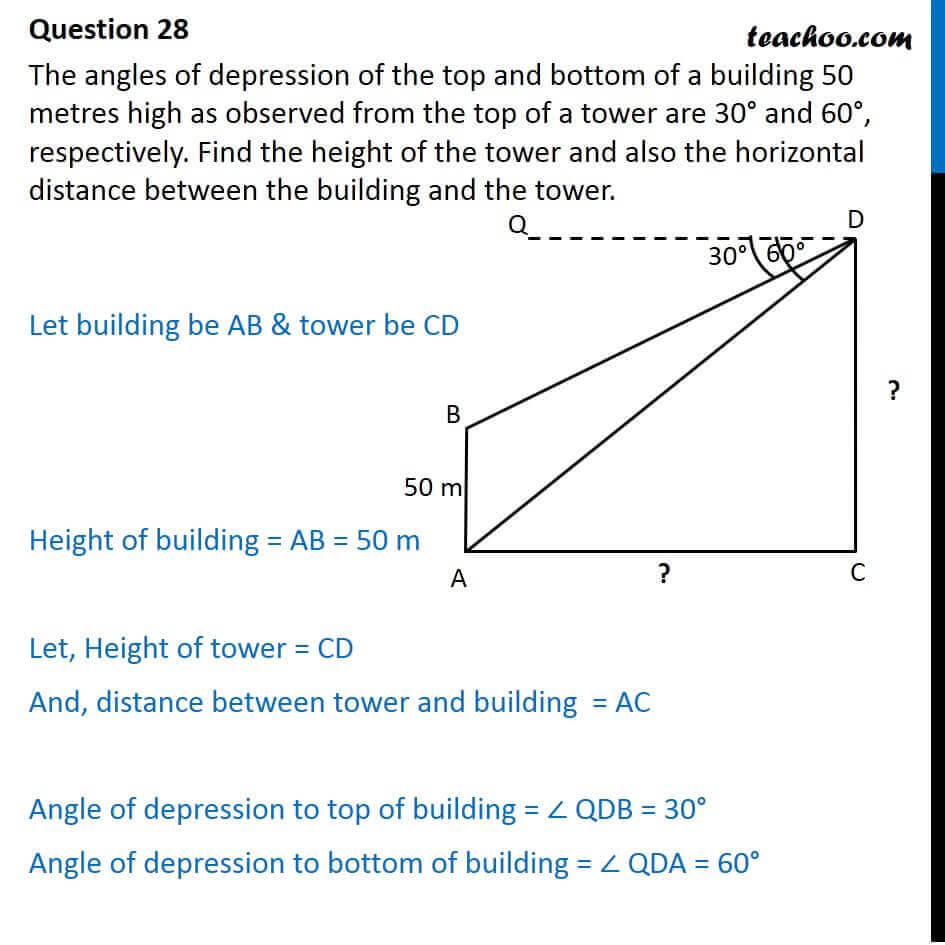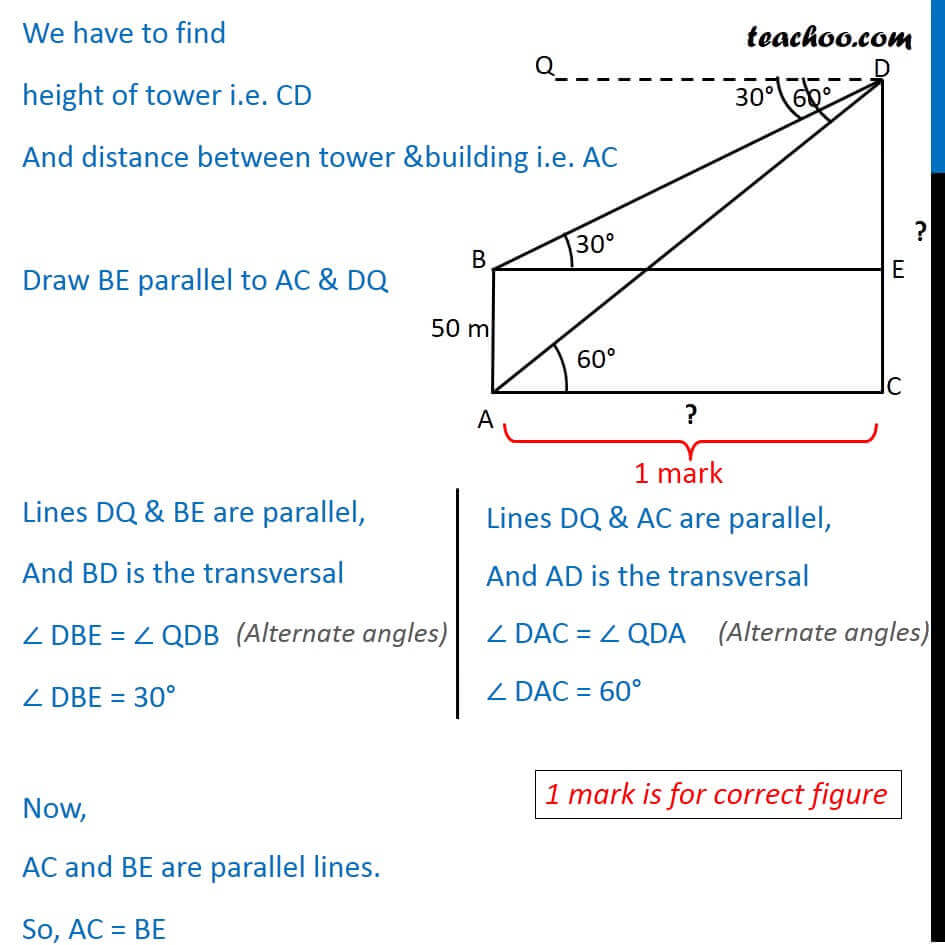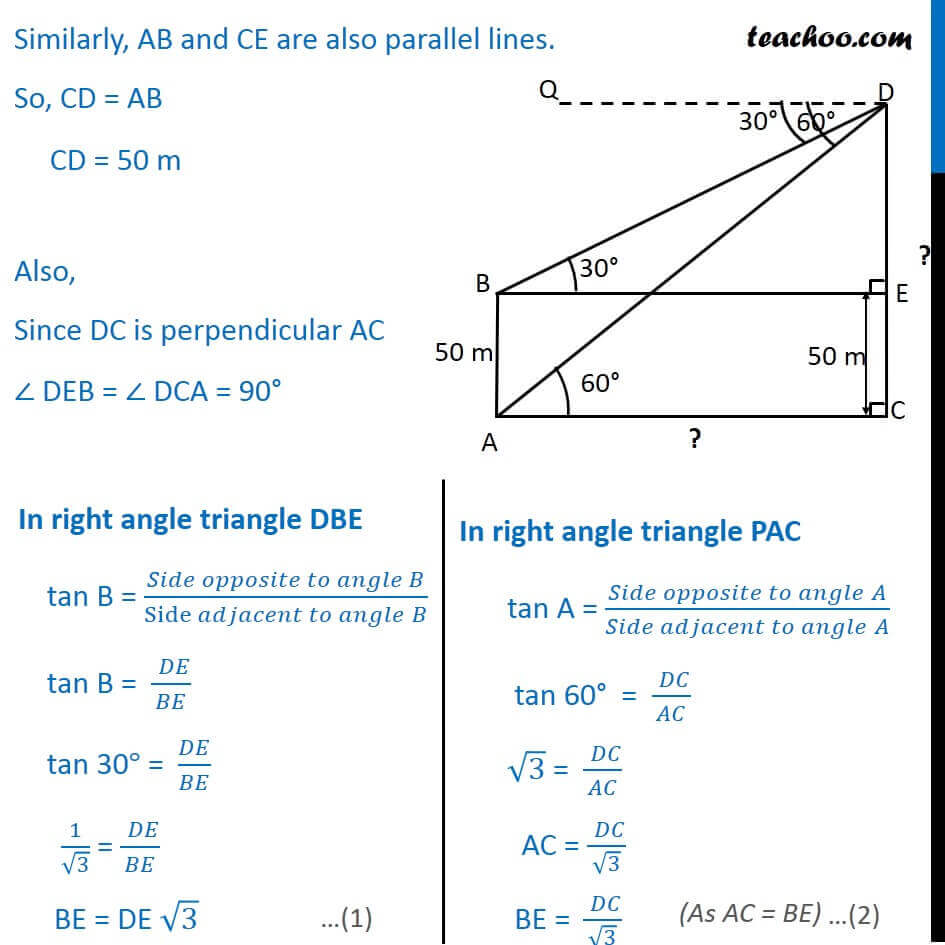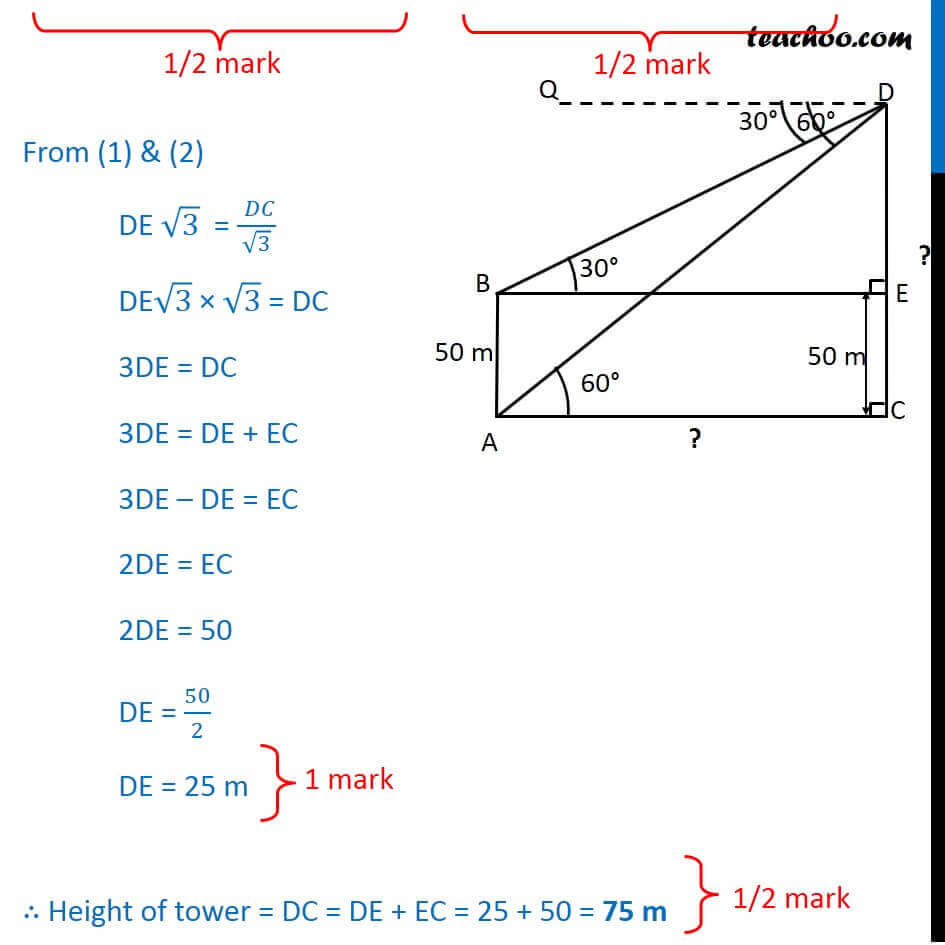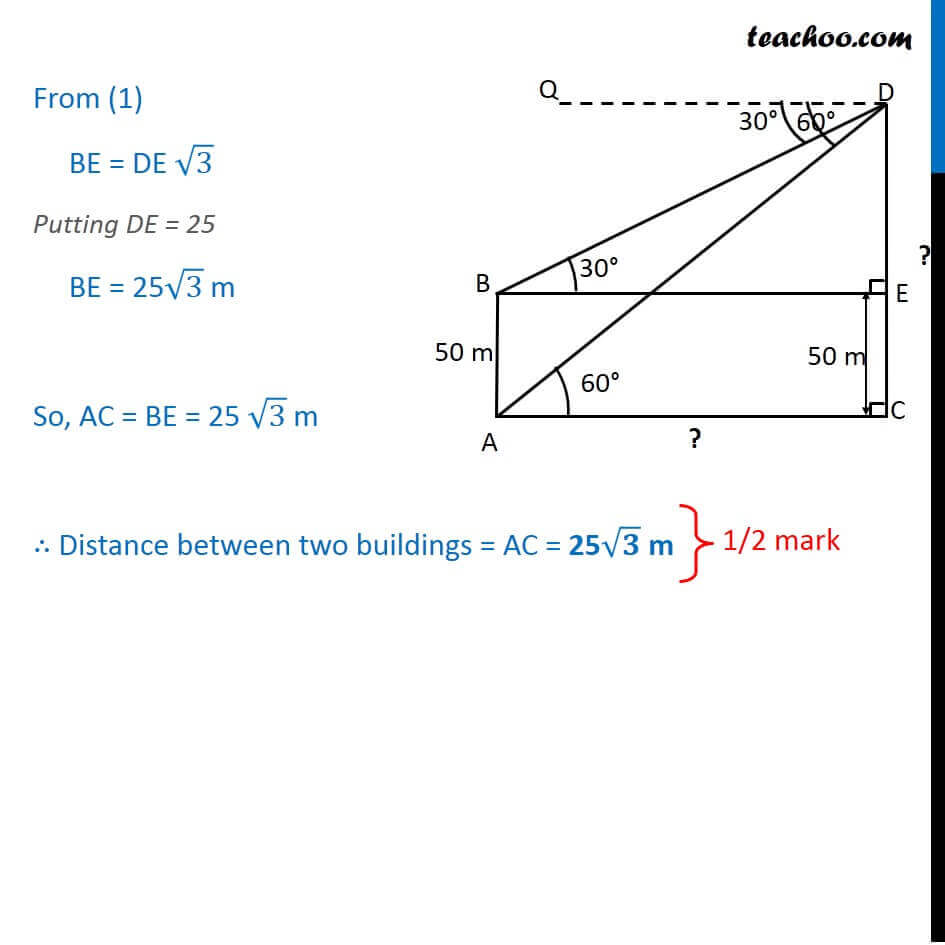Learn in your speed, with individual attention - Teachoo Maths 1-on-1 Class

### Transcript

Question 28 The angles of depression of the top and bottom of a building 50 metres high as observed from the top of a tower are 30 and 60 , respectively. Find the height of the tower and also the horizontal distance between the building and the tower. Let building be AB & tower be CD Height of building = AB = 50 m Let, Height of tower = PC And, distance between tower and building = AC Angle of depression to top of building = QDB = 30 Angle of depression to bottom of building = QDA = 60 We have to find height of tower i.e. CD And distance between tower &building i.e. AC Draw BE parallel to AC & DQ Lines DQ & BE are parallel, And BD is the transversal DBE = QDB DBE = 30 Now, AC and BE are parallel lines. So, AC = BE Lines DQ & AC are parallel, And AD is the transversal DAC = QDA DAC = 45 Similarly, AB and CE are also parallel lines. So, CD = AB CD = 50 m Also, Since DC is perpendicular AC DEB = DCA = 90 In right angle triangle DBE tan B = ( )/(Side " " ) tan B = (" " )/ tan 30 = / 1/ 3 = (" " )/ BE = DE 3 In right angle triangle PAC tan A = ( " " )/( " " ) tan 60 = (" " )/ 3 = (" " )/ AC = (" " )/ 3 "BE = " (" " )/ 3 From (1) & (2) DE 3 = (" " )/ 3 DE 3 3 = DC 3DE = DC 3DE = DE + EC 3DE DE = EC 2DE = EC 2DE = 50 DE = 50/2 DE = 25 m Height of tower = DC = DE + EC = 25 + 50 = 75 m From (1) BE = DE 3 Putting DE = 25 BE = 25 3 m So, AC = BE = 25 3 m Distance between two buildings = AC = 25 m